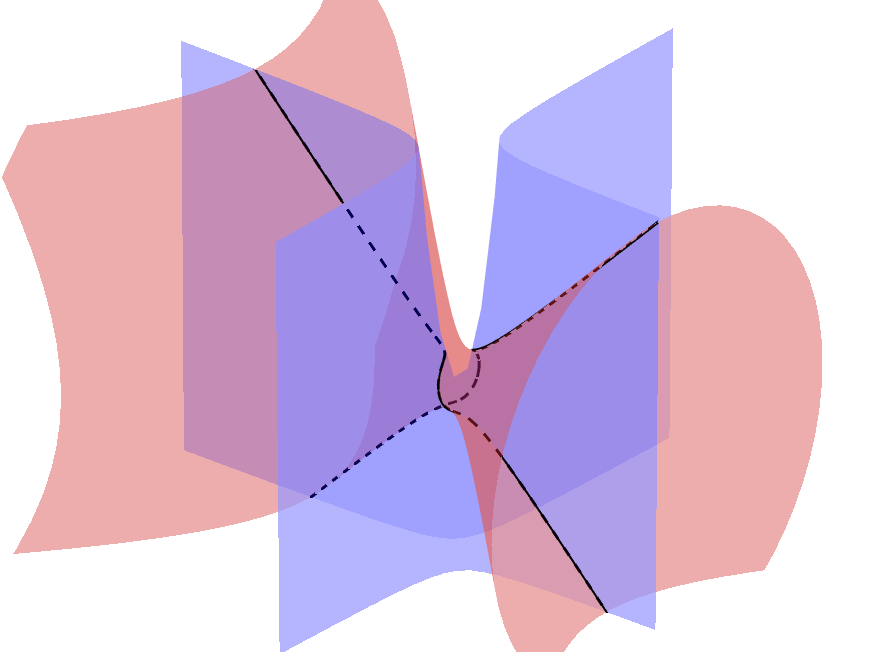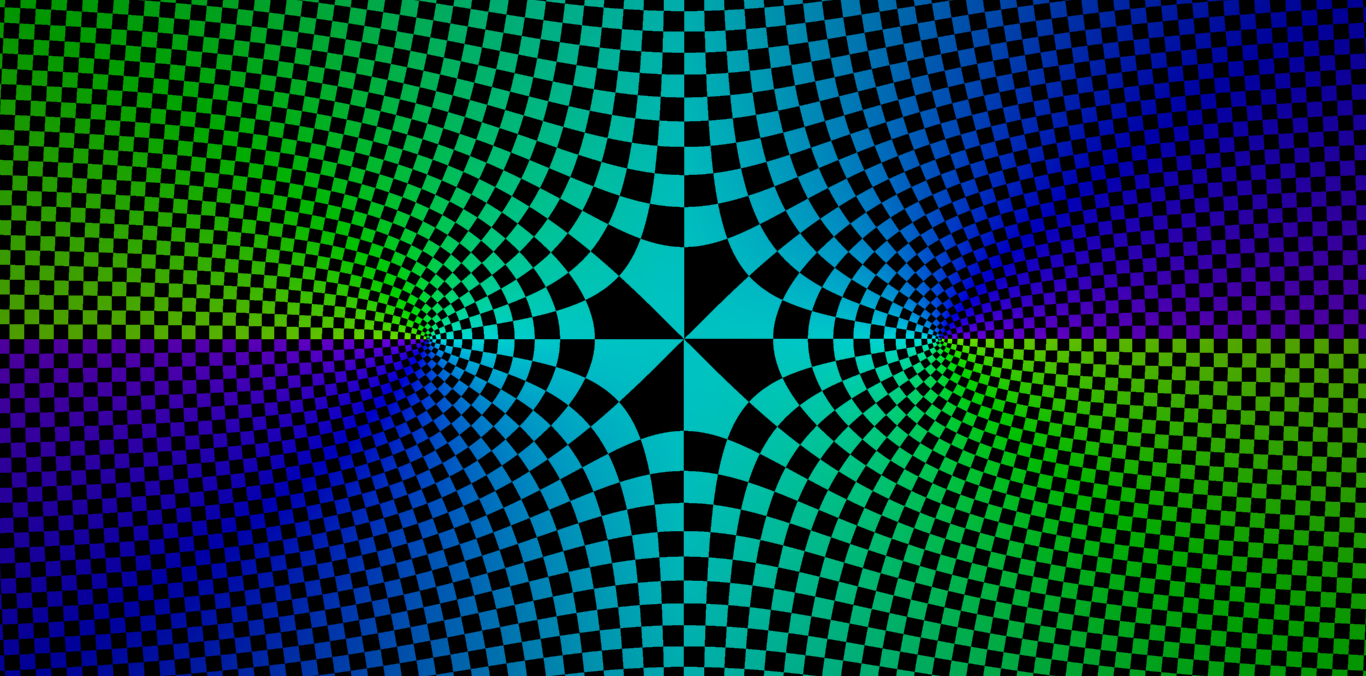NIKOLA UBAVIĆSrpski

# Complex sphere

It is well known that the set of all points $$(x, y)\in\mathbb{R}^2$$ which satisfy the equation $x^2+y^2-1=0\tag{1}$ forms a circle (i.e. a one-dimensional sphere $$S^1$$ embedded in $$\mathbb{R}^2$$) centred at the origin and with a radius $$1$$.

Sphere $$S^1$$ without a single point $$\left(0,1\right)$$ can be also presented as a continuous image of $$\mathbb{R}$$ by mapping $\phi^+\left(t\right)=\left(\frac{2t}{1+t^2},\frac{t^2-1}{1+t^2}\right)\tag{2}.$ Similarly, a sphere $$S^1$$ without a point $$\left(0,-1\right)$$ can be presented as a continuous image of a set $$\mathbb{R}$$ by mapping$\phi^-\left(t\right)=\left(\frac{2t}{1+t^2},\frac{t^2-1}{1+t^2}\right).$

In fact, every finite-dimensional sphere $$S^n$$ can be similarly "covered"1 with two mappings: $\phi^\pm\left(t_1,\dots, t_n\right)=\frac{1}{1+t_1^2 +\cdots+t_n^2}\left(2t_1,\dots, 2t_n,\pm\left(-1+t_1^2 +\cdots+t_n^2\right)\right).\tag{3}$

Mappings (3) and their inverse mappings $(\phi ^{\pm}) ^{- 1}\left(x_1, ..., x_{n+1}\right) =\frac{1}{1\pm x_{n+1}}\left(x_1,\dots, x_n\right),$ are called stereographic projections. Stereographic projection $$\phi^+$$ is constructed in that way so that the point, its image and the point $$(0,\dots, 0,1)$$ are collinear points, as shown in Figure 1. Stereographic projection $$\phi^-$$ is constructed in that way so that the point, its image and the point $$(0,\dots, 0,-1)$$ are collinear pointsFigure 1. Stereographic projection $$\phi^{+}$$ with $$S^2$$ on $$\mathbb{R}^2$$.

Let us return to equation (1). The following question naturally arises: What complex variety is determined by the polynomial (1)? In other words, what geometric object $$F\subset\mathbb{C}^2$$ is determined by the zeros of the complex polynomial $$P(z_1,z_2)=z_1^2+z^2-1.$$

If $$P(z_1, z_2) = 0$$ for complex numbers $$z_1 = x+iy$$ and $$z_2 = z+iw$$, then \begin{aligned}\Re\left(z_1^2+z_2^2\right)&=1, \\ \Im\left(z_1^2+z_2^2\right) &= 0,\end{aligned} and is $x^2-y^2+z^2-w^2=1,\\xy+zw=0.\tag{5}$

Although $$F$$ is a subset of $$\mathbb{C}^2$$, we can see it as a subset of $$\mathbb{R}^4$$. Thus, we see that equations (5) give two independent conditions for the coordinates of the set $$F$$ in 4-dimensional space. Therefore $$F$$ is a surface2.

As we don't have intuition for four dimensions, we can see this surface only through two-dimensional and three-dimensional sections of $$\mathbb{R}^4$$. In the rest of this text, we will determine the sections of the surface $$F$$ with three-dimensional space $$w=\lambda$$ for $$\lambda\in\mathbb{R}$$. In this case equations (5) become $x^2-y^2+z^2=1+\lambda^2\tag{6}$ $xy = -\lambda z.\tag{7}$ so we see that $$F\cap\left\{w =\lambda\right\}$$ is in the intersection of a hyperbolic paraboloid and a hyperboloid of one sheet. One such intersection is shown in Figure 2.Figure 2. Cross section of hyperboloid of one sheet and hyperbolic paraboloid.

To find the parameterization of this intersection, we can express $$z$$ from the equation (7), when $$\lambda\ne 0$$. Substituting this into equation (6) we get $$x^2+y^2\left(-1+x^2 /\lambda^2\right) = 1 +\lambda^2$$. Solving now for $$y$$ gives us $y =\pm\sqrt{\frac{\lambda^2}{x^2-\lambda^2} -\lambda^2}.$ It follows from equation (7) that $z=\mp\sqrt{\frac{x^2}{x^2-\lambda^2} -x^2}.$ This is how we expressed $$y$$ and $$z$$ coordinates in terms of $$x$$ coordinate, so we can parameterize a curve $$F\cap\left\{w =\lambda\right\}$$ as follows: ${\bold r}\left(t\right) =\left(t,\pm\sqrt{\frac{\lambda^2}{t^2-\lambda^2} -\lambda^2},\pm\sqrt{\frac{t^2}{t^2-\lambda^2} -t^2}\right).\tag{8}$

In the case when $$\lambda=0$$, equation (7) represents the union of two planes, so the intersection $$F\cap\left\{w = 0\right\}$$ is the union of circle and hyperbola (see Figure 3). The circle that lies in that intersection is actually the circle that polynomial $$x^2+y^2-1$$ defines in the real plane.Figure 3. Left figure shows a hyperbolic paraboloid $$xy+zw=0$$. The right image shows planes $$x=0$$ and $$y=0$$.

We can use (8) to find the parameterization of the projection of the whole surface $$F$$ to the three-dimensional space. It is enough to parameter $$\lambda$$ use as a variable. In that way, we get the following parameterization3 ${\bold r}\left(u, v\right) =\left(u,\pm\sqrt{\frac{v^2}{u^2-v^2} -v^2},\pm\sqrt{\frac{u^2}{u^2-v^2}-u^2}\right).\tag{9}$ This surface is shown in Figure 4.Figure 4. Projection of $$F$$ on the subspace $$w = 0$$.

Part of the surface $$F$$ can also been seen as a graph of one branch of the complex function $$z_2=\sqrt{1-z_1^2}$$ as is shown in Figure 4. In that figure, each point $$z_1$$ is colored with the color of the point $$z_2=f(z_1)$$ by standard4 HSV complex plane coloring.Figure 5. Graph of a complex function $$z_2=\sqrt{1-z_1^2}$$.

In this way, the image of the variety is determined $$F$$ in projection $$\left(x, y, z, w\right)\mapsto\left(x, y, z\right)$$. Due to the symmetry of equations (6) and (7), it is clear that the same surface (up to the relabeling of coordinates) will be obtained in the projection $$\left(x, y, z, w\right)\mapsto\left(x, z, w\right)$$. However, with projections $$\left(x, y, z, w\right)\mapsto\left(x, y, w\right)$$ and $$\left(x, y, z, w\right)\mapsto\left(y, z, w\right)$$ we get completely different surfaces. For example, in the first case we get the parameterization: ${\bold r}\left(u, v\right) =\left(u,\pm\sqrt{v^2-\frac{v^2}{u^2-v^2}},\pm\sqrt{u^2-\frac{u^2}{u^2-v^2}}\right).$

Finally, notice that circle $$S^1$$ embedded in the complex plane $$\mathbb{C}$$ can't be the zero set of any polynomial from $$\mathbb{C}[z_1]$$, although it is zero set in $$\mathbb{R}^2$$ of a polynomial from $$\mathbb{R}[x_1, x_2]$$.

1. Except they are mappings $$\phi ^{\pm}$$ and their inversions are continuous, these mappings are also smooth. It follows that every sphere $$S^n$$ is smooth real manifold with an atlas of two maps.
2. The notion of a real surface corresponds to the notion of a complex curve. So a polynomial $$x^2+y^2-1 = 0$$ determines the complex curve in $$\mathbb{C}^2$$, just as it determines the real curve in $$\mathbb{R}^2$$.
3. This parameterization is not suitable for drawing in programs due to computational error made during sharing as well as due to unusual domain shape (known surface drawing programs such as Wolphram Mathematica, GeoGebra, gnuplot, etc... can't overcome these problems on their own).
4. In this coloring, points are assigned a hue depending on the argument of that point, and the brightness of that tone depending on the modulus of that point.